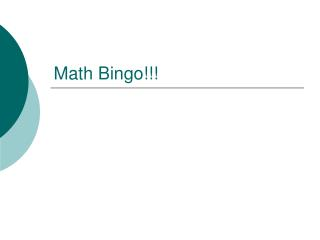# Math Bingo!!! - PowerPoint PPT PresentationDownload PresentationMath Bingo!!!

Math Bingo!!!Download Presentation## Math Bingo!!!

- - - - - - - - - - - - - - - - - - - - - - - - - - - E N D - - - - - - - - - - - - - - - - - - - - - - - - - - -
##### Presentation Transcript

1. Math Bingo!!!

2. Rules: • Fill in your Bingo card • You can only use each person’s name twice • Everyone must do the problems on a sheet of paper that will be turned in • If that student gets the answer correct, then you can mark his/her name off on the Bingo card. • If that student misses the answer, then I will continue calling on students until it is correct. • Bingo winners will get a sucker!!!

3. Solve for the variable 1. -12x = 72 2. 9x = -36 3. 4. Answers: 1) -6 2) -4 3) 77 4) -27/6

4. Solve for the variable 5. 6. 7. x + 3.2 = -4.8 Answers: 5) 26/15 6) -23 7) -8.0 or -8

5. Solve for x 8. 2x – 6 = 14 9. 10 – 3y = 40 10. 3x + 5x = -24 Answers: 8)10 9) -10 10) -3

6. Solve for x 11. 2(x – 4) = 18 12. 8y – (8 + 6y) = 20 13. ½(4x – 10) = 35 14. ¾(x + 9) = 15 15. 5c + (-8c) = 36 Answers: 11) 13 12) 14 13) 20 14) 11 15) -12

7. Simplify • 8x + 4y – (4x – 5y) • 7r – (s + 2r) – 4s • 8y³ for y = 3 Answers: 16) 4x + 9y 17) 5r – 5s 18) -8 19) 216

8. Homework • Worksheet #1 • Review Day tomorrow • Quiz #1 Friday